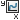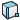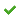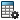# Plotting Forces

You can plot forces on an assembly due to motors, forces, friction, or contact.

1. From a Motion Analysis study, click Results and Plots(MotionManager toolbar).
2. In the PropertyManager, under Result, for Category, select Forces.
3. For Subcategory and its corresponding features, select from the following:
Subcategory FeaturesMotor Force Linear motor
Reaction Force Force
Friction Force Select from the following:
• Two part faces that have contact to compute the friction force exerted by the first selected component on the second component.
• A mate and an optional face of a part attached to the mate to compute the friction force exerted on the face at the mate.
If you do not select a face, the force is computed at the first face that defines the mate.
• A curve-to-curve contact feature to compute the friction force exerted by the first selected component defining the curve-to-curve contact on the second component.
Contact Force Select from the following:
• Two part faces that have contact
• A curve-to-curve contact feature
4. For Select result component, select an option:
• Magnitude to resolve the magnitude of the force vector with respect to global coordinates.
• X Component, Y Component, or Z Component to calculate the magnitude of the force projected to the selected direction with respect to global coordinates.
Optionally, you can select a reference partto plot the result relative to the coordinates of that part.
5. Select the Plot Results options you require, and click.
The result appears in the MotionManager tree.
6. Click Calculate(MotionManager toolbar).